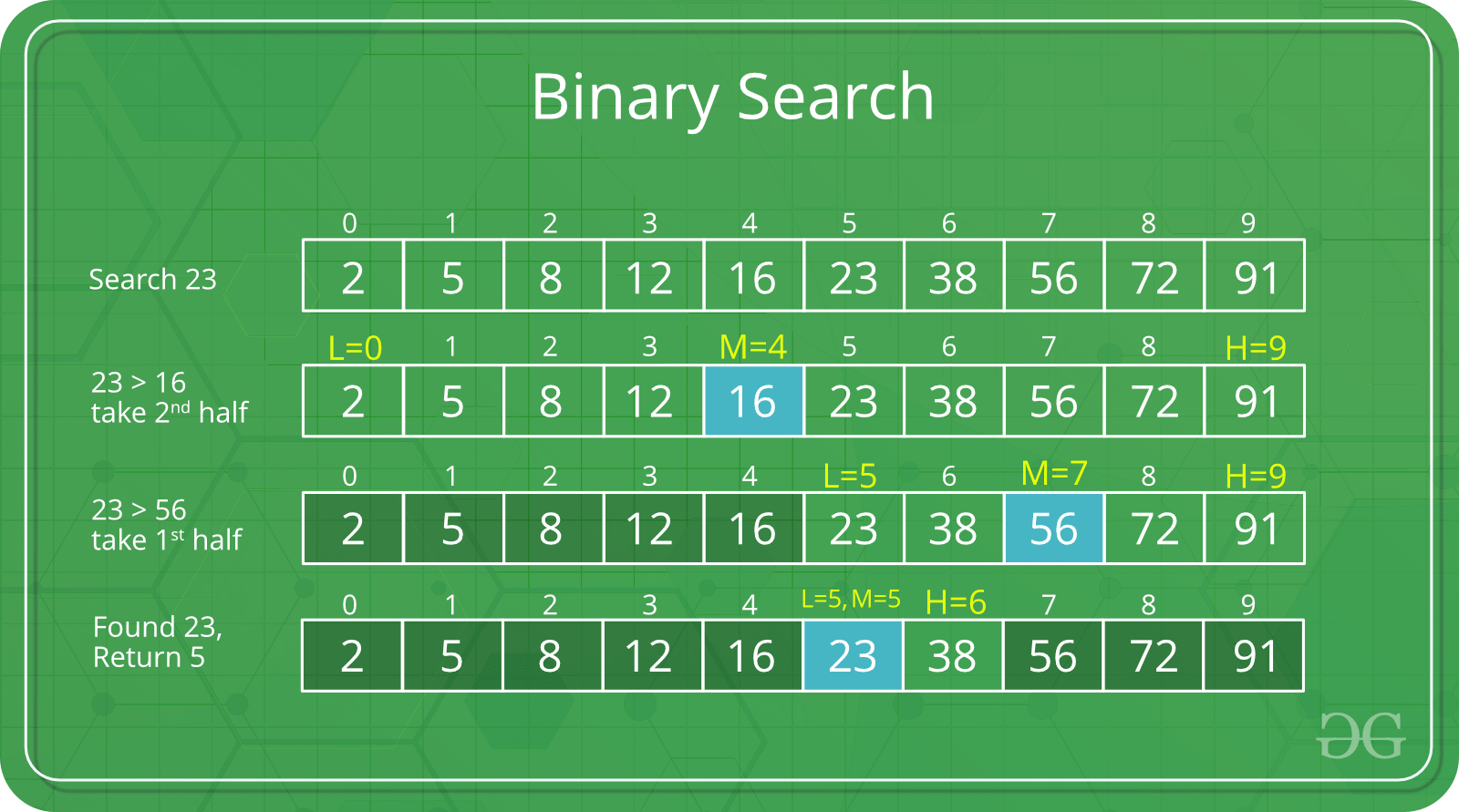Skip to content
Related Articles
Binary Search in Scala
• Last Updated : 03 Jul, 2020

Binary Search is an algorithm that helps us find an element in a sorted array in O(log n) time. Its algorithm works on the principle of Divide and Conquer and it works only when the available data is sorted.
Now, when we are using binary search three situation arise:

1. If the middle element is the element to be searched, we return the index of the middle element.
2. If the middle element is smaller than the element to be searched, we search in the sub-array to the right (Mid to End) in the same way (Get the new middle and check all the three cases again). We search in the right sub-array because the data is sorted, thus all elements before middle element will also be either less than or equal to the middle element.
3. If the middle element is greater than the element to be searched, we search in the sub-array to the left(Start to Mid).

This process is continued until either we find the element to be searched or the size of the sub-array reduces to zero.

Example:There are three ways to perform Binary Search in Scala.

### Recursive Approach

In recursive approach, we recursively call for the implemented binary search algorithm with updated values of start and end until either we match the middle element with the element to be searched or the array size reduces to zero. Below is the code for the recursive approach of Binary Search in Scala.

 `// Scala code for Recursive Binary Search`` ` `// Creating object``object` `GFG{`` ` `// Creating a recursive  Binary Search function``def` `RecursiveBinarySearch(arr``:` `Array[Int],``                          ``Element``_``to``_``Search``:` `Int)``                         ``(low``:` `Int ``=` `0``,``                          ``high``:` `Int ``=` `arr.length - ``1``)``:` `Int ``=` `{``     ` `    ``// If element not found                               ``    ``if` `(low ``>` `high) ``        ``return` `-``1``     ` `    ``// Getting the middle element``    ``var` `middle ``=` `low + (high - low) / ``2``     ` `    ``// If element found``    ``if` `(arr(middle) ``==` `Element``_``to``_``Search)``        ``return` `middle``     ` `    ``// Searching in the left half``    ``else` `if` `(arr(middle) ``>` `Element``_``to``_``Search)``        ``return` `RecursiveBinarySearch(arr, ``               ``Element``_``to``_``Search)(low, middle - ``1``)``     ` `    ``// Searching in the right half``    ``else``        ``return` `RecursiveBinarySearch(arr, ``               ``Element``_``to``_``Search)(middle + ``1``, high)``}`` ` `// Creating main function``def` `main(args``:` `Array[String]){``     ` `    ``// Calling the binary search function and``    ``// storing its result in index variable``    ``var` `index ``=` `RecursiveBinarySearch(Array(``1``, ``2``, ``3``, ``4``, ``55``, ``                                            ``65``, ``75``), ``4``)(``0``, ``6``);``     ` `    ``// If value not found ``    ``if``(index ``==` `-``1``)``       ``print(``"Not Found"``)``         ` `    ``// Else print the index where ``    ``// the value is found``    ``else``       ``print(``"Element found at Index "` `+ index)``}``}`

Output

`Element found at Index 3`

### Iterative approach

In iterative approach, we run a while loop until we either find the element to be searched or the array size reduces to zero. Below is the code for iterative approach of Binary Search in Scala.

 `// Scala code for Iterative Binary Search`` ` `// Creating object``object` `GFG{`` ` `// Creating Binary Search function``// Accepting the passed array and ``// element to be searched``def` `IterativeBinarySearch(arr``:` `Array[Int], ``                          ``Element``_``to``_``Search``:` `Int)``:` `Int ``=``{``     ` `    ``// Creating start variable to``    ``// point to the first value``    ``var` `low ``=` `0``     ` `    ``// Creating end variable to ``    ``// point to the last value``    ``var` `high ``=` `arr.length - ``1``     ` `    ``// Finding the value in the ``    ``// array iteratively``    ``while` `(low ``<=` `high)``    ``{``         ` `        ``// Getting middle element    ``        ``var` `middle ``=` `low + (high - low) / ``2``         ` `        ``// If element found in the middle index``        ``if` `(arr(middle) ``==` `Element``_``to``_``Search)``            ``return` `middle``         ` `        ``// Searching in the first half``        ``else` `if` `(arr(middle) ``>` `Element``_``to``_``Search)``            ``high ``=` `middle - ``1``         ` `        ``// Searching in the second half  ``        ``else``            ``low ``=` `middle + ``1``    ``}``     ` `    ``// If value not found in the ``    ``// entire array , return -1 ``    ``-``1``}`` ` `// Creating main function``def` `main(args``:` `Array[String])``{``     ` `    ``// Calling the binary search function and``    ``// storing its result in index variable``    ``var` `index ``=` `IterativeBinarySearch(Array(``1``, ``2``, ``3``, ``4``, ``55``,``                                            ``65``, ``75``), ``65``);``     ` `    ``// If value not found ``    ``if``(index ``==` `-``1``)``       ``print(``"Not Found"``)``         ` `    ``// Else print the index where ``    ``// the value is found``    ``else``       ``print(``"Element found at Index "` `+ index)``}``}`

<Output

`Element found at Index 5`

### Pattern Matching And Functional Programming Approach

In this, we first match the middle element with the element to be searched. If the element is present, we return the index of it. Otherwise, we keep calling the created function with the updated parameters. Below is the code for the approach:

 `// Scala code for Iterative Binary Search`` ` `// Creating object``object` `GFG{``     ` `// Using the functional programming approach``def` `FunctionalBinarySearch(arr``:` `Array[Int], ``                           ``Element``_``to``_``Search``:` `Int)``:` `Int ``=``{ ``    ``def` `BinarySearch(arr``:` `Array[Int],``                     ``Element``_``to``_``Search``:` `Int, ``                     ``low``:` `Int, high``:` `Int)``:` `Int ``=``    ``{``             ` `        ``// If element not found``        ``if` `(low ``>` `high)``            ``return` `-``1``             ` `        ``// Getting middle index``        ``var` `middle ``=` `low + (high - low) / ``2``             ` `        ``// Pattern matching``        ``arr ``match``        ``{``                 ` `            ``// If element found , return the index``            ``case``(arr``:``Array[Int]) ``if` `(arr(middle) ``==``                                     ``Element``_``to``_``Search) ``=>` `                                     ``middle ``                 ` `            ``// Call the function for the second half``            ``case``(arr``:``Array[Int]) ``if` `(arr(middle) ``<` `                                     ``Element``_``to``_``Search) ``=>` `                                     ``BinarySearch(arr, ``                                     ``Element``_``to``_``Search,``                                     ``middle + ``1``, high)``                 ` `            ``// Call the function for the first half ``            ``case``(arr``:``Array[Int]) ``if` `(arr(middle) ``>` `                                     ``Element``_``to``_``Search) ``=>` `                                     ``BinarySearch(arr, ``                                     ``Element``_``to``_``Search,``                                     ``low, middle - ``1``)``        ``}``    ``} ``         ` `    ``// Calling the Binary Search function``    ``BinarySearch(arr, Element``_``to``_``Search, ``0``, arr.length - ``1``)``}``     ` `// Creating main function``def` `main(args``:` `Array[String]){`` ` `    ``// Calling the binary search function and ``    ``// storing its result in index variable``    ``var` `index ``=` `FunctionalBinarySearch(Array(``1``, ``2``, ``3``, ``4``, ``55``,``                                             ``65``, ``75``), ``44``);`` ` `    ``// If value not found ``    ``if``(index ``==` `-``1``)``    ``print(``"Element not found"``)``         ` `    ``// Else print the index where ``    ``// the value is found``    ``else``    ``print(``"Element found at Index "` `+ index)``}``}`

Output

```Element not found
```

My Personal Notes arrow_drop_up